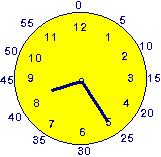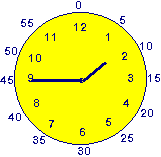Telling Time - Subrtacting Time

Telling Time - Clocks

How do you subtract time?

When subtracting time you do not look at each digit. You look at the minutes as a whole. In the problem below you can not subtract 52 minutes   from 15 minutes (15 - 52 = ???), because 15 is smaller. You must borrow.

 5 hours 15 minutes - 1 hour 52 minutes ???????????????
```How do you do this? You go to your hours and take 1 away. (5 - 1 = 4hours)
You now have 4 hours.```
```An hour equals 60 minutes. You add the 60 minutes to the 15 minutes.
(60 + 15 = 75 minutes) This gives you 75 minutes. Your problem now looks like this.```
 4 hours 75 minutes - 1 hour 52 minutes
```Now you are able to subtract.
```
 4 hours 75 minutes - 1 hour 52 minutes 3 hours 23 minutes
`The answer is 3 hours and 23 minutes.`
`Remember this:`
• ```Borrowing and carrying in regular math means exchanging 10  ones for a ten
(or 10 tens for a hundred, etc.).```
• ```Borrowing and carrying in time math mean exchanging 60 minutes for an hour.
```

You follow the same rules when you are trying to figure out what time it was so many hours and minutes ago?

Example:What time was it 3 hours and 45 minutes ago?

Turn this into a subtraction problem. The clock says it is 8:25. Subtract 3:45 from 8:25.

 8:25  --->regroup--> - 3:45
 7:85 - 3:45

Now you are able to subtract. The answer is 4:40. 3 hours and 45 minutes before 8:25 was 4:40.

Example 2What time was it 3 hours and 50 minutes ago?

Turn this into a subtraction problem. The clock says it is 1:45. Subtract 3:50 from 1:45.

 1:45  --->regroup--> - 3:50
 2:105 - 3:50

It is easy to subtract the minutes 105 - 50 = 55. The hours can not be subtracted
2 - 3 = ??

You must look at the clock when you encounter a problem like this one. The hour hand is on the one. Take away 3 hours from this. When you back the clock up 3 hours from 1 you get 10. Add this to your minutes.